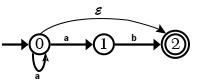# What is the difference between DFA and NFA in compiler design?

Deterministic Finite Automata (DFA)

Deterministic means that on each input there is one and only one state to which the automata can have the transition from its current state. In deterministic finite automata, the head can move only in one direction to scan the input tape symbols. But in the case of two-way, finite automata on scanning an input symbol the head of the tape may move in right or left from its current position.

There are two ways to represent Deterministic finite Automata −

• Transition Diagram

It is a directed graph or flow chart having states and edges. A strong path through a transition graph is a sequence of edges forming a path starting at some start state and ending at a final state.

• Transition Table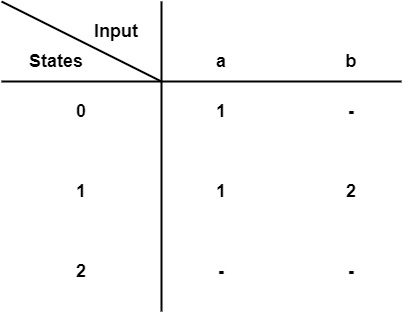Finite Automata can be represented by 5 tuples (Q, Σ, δ, q0, F)

• Q is a finite non-empty set of states.
• Σ is a finite set of input symbols.
• 𝛿 is the transition function.
• q0 ∈ Q is the initial state.
• F ⊆ Q is the set of final states.

Non-Deterministic Finite Automata (NFA)

Non-Deterministic means that there can be several possible transitions. So, the output is non-deterministic for a given input. NFA can also be represented as a nondeterministic finite state machine. In NFA, the transitions are not specifically decided by their input symbol or source state. The reading of input symbols is not needed for each single state transition.

Example1− Draw NFA for the Regular Expression a(a + b) *ab

Solution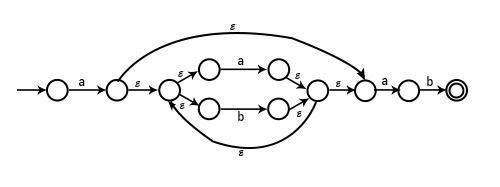Example2− Draw NFA for a + b + ab

Solution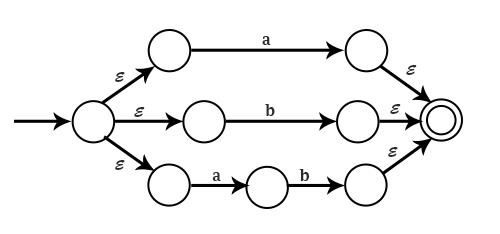Let us see the comparison between DFA and NFA.

DFANFA
Every from one state to other is unique and deterministic.There can be multiple transitions for input, i.e., non-deterministic.
Null transition (ε) is not allowed.Null transition (ε) are allowed means a transition from the current state to the next state without any input.
Transition function− δ− Q x Σ → QTransition function− δ− Q x Σ → 2Q
It requires less memory as transitions and states are lessIt requires more memory.
Example of DFA Each state has unique input symbol Σ = {a, b} outgoing from it.Example of NFA As there are multiple transitions of input symbol 'a' on state 0. & no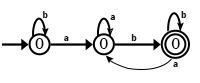transition from state 2, i.e., nondeterministic.